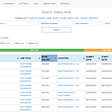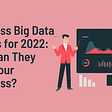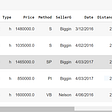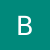# Pre Requisite

• Azure account
• Azure storage
• Azure machine learning services

# Code Sample

• Using notebook code to perform responsible ai features
• Explainability
• Fairness
• and more

# library installation

`!pip install raiwidgets==0.9.2!pip install fairlearn==0.7.0`
• Check the versions
`!pip show fairlearn!pip show raiwidgets`
• Now the actual code
• Load the data from dataset or from file
`from raiwidgets import ExplanationDashboardfrom azureml.core import Datasetfrom azureml.data.dataset_factory import DataType# create a TabularDataset from a delimited file behind a public web url and convert column "Survived" to booleanweb_path ='https://dprepdata.blob.core.windows.net/demo/Titanic.csv'titanic_ds = Dataset.Tabular.from_delimited_files(path=web_path, set_column_types={'Survived': DataType.to_bool()})# preview the first 3 rows of titanic_dstitanic_ds.take(3).to_pandas_dataframe()from azureml.core import Workspace, Datasetimport pandas as pdimport numpy as npdf = pd.read_csv('Titanic.csv')df.head()df['id'] = df[['Name']].sum(axis=1).map(hash)titanic_features = df.copy()titanic_labels = titanic_features.pop('Survived')df.drop('Name', axis=1, inplace=True)from sklearn.preprocessing import LabelEncoder, OneHotEncoderimport sklearn as skdf1 = pd.get_dummies(df)y = df1['Survived']X = df1X = X.drop(columns=['Survived'])X['Age'] = X['Age'].fillna(0)X = X.dropna()import pandas as pdimport seaborn as snimport matplotlib.pyplot as pltfrom sklearn.preprocessing import StandardScalerfrom sklearn.linear_model import LogisticRegressionfrom tqdm import tqdmfrom sklearn import preprocessingfrom sklearn.model_selection import train_test_splitX_train, X_test, y_train, y_test = train_test_split(X, y, test_size=0.33, random_state=42)import sklearn as skfrom sklearn.linear_model import LogisticRegressionLR = LogisticRegression(random_state=0, solver='lbfgs', multi_class='ovr').fit(X, y)LR.predict(X.iloc[460:,:])round(LR.score(X,y), 4)y_pred = LR.predict(X_test)from sklearn.metrics import roc_auc_scorefrom sklearn.metrics import f1_scorefrom sklearn import metricsprint(metrics.classification_report(y_test, y_pred))from sklearn.datasets import load_breast_cancerfrom sklearn import svm# Explainers:# 1. SHAP Tabular Explainer#from interpret.ext.blackbox import TabularExplainerfrom interpret.ext.blackbox import TabularExplainerclasses = X_train.columns.tolist()explainer = TabularExplainer(LR,                              X_train,                              features=X.columns,                              classes=['Sex_male', 'Sex_female'])from interpret.ext.blackbox import MimicExplainer# you can use one of the following four interpretable models as a global surrogate to the black box modelfrom interpret.ext.glassbox import LGBMExplainableModelfrom interpret.ext.glassbox import LinearExplainableModelfrom interpret.ext.glassbox import SGDExplainableModelfrom interpret.ext.glassbox import DecisionTreeExplainableModel# "features" and "classes" fields are optional# augment_data is optional and if true, oversamples the initialization examples to improve surrogate model accuracy to fit original model.  Useful for high-dimensional data where the number of rows is less than the number of columns. # max_num_of_augmentations is optional and defines max number of times we can increase the input data size.# LGBMExplainableModel can be replaced with LinearExplainableModel, SGDExplainableModel, or DecisionTreeExplainableModelexplainer = MimicExplainer(LR,                            X_train,                            LGBMExplainableModel,                            augment_data=True,                            max_num_of_augmentations=10,                            features=X.columns,                            classes=['Sex_male', 'Sex_female'])global_explanation = explainer.explain_global(X_test)from raiwidgets import ExplanationDashboardExplanationDashboard(global_explanation, LR, dataset=X_test, true_y=y_test)`
`# Sorted SHAP valuesprint('ranked global importance values: {}'.format(global_explanation.get_ranked_global_values()))# Corresponding feature namesprint('ranked global importance names: {}'.format(global_explanation.get_ranked_global_names()))# Feature ranks (based on original order of features)print('global importance rank: {}'.format(global_explanation.global_importance_rank))# Note: Do not run this cell if using PFIExplainer, it does not support per class explanations# Per class feature namesprint('ranked per class feature names: {}'.format(global_explanation.get_ranked_per_class_names()))# Per class feature importance valuesprint('ranked per class feature values: {}'.format(global_explanation.get_ranked_per_class_values()))# Print out a dictionary that holds the sorted feature importance names and valuesprint('global importance rank: {}'.format(global_explanation.get_feature_importance_dict()))sex = df['Sex']sex.value_counts()y_true = y_testlen(y_test)len(y_pred)sensitivefeatures = X_test[['Sex_male', 'Sex_female']]sensitivefeaturesprint ("Confusion Matrix:")print (metrics.confusion_matrix(y_test, y_pred))gm = MetricFrame(metrics=accuracy_score, y_true=y_test, y_pred=y_pred, sensitive_features=sensitivefeatures)print(gm.overall)print(gm.by_group)from fairlearn.metrics import selection_ratesr = MetricFrame(metrics=selection_rate, y_true=y_true, y_pred=y_pred, sensitive_features=sensitivefeatures)`
• Fairness
`from raiwidgets import FairnessDashboard# A_test contains your sensitive features (e.g., age, binary gender)# y_true contains ground truth labels# y_pred contains prediction labelsFairnessDashboard(sensitive_features=sensitivefeatures,                  y_true=y_test.tolist(),                  y_pred=y_pred)`

Originally published at https://github.com.

--

--

--

## More from Analytics Vidhya

Analytics Vidhya is a community of Analytics and Data Science professionals. We are building the next-gen data science ecosystem https://www.analyticsvidhya.com

## Cities with Highest (rent-adjusted) Salaries## COVID-19 Cases By The End Of June | A Time Series Forecasting Using FB Prophet## Writing your own Scikit-learn classes — for beginners.## Data warehouse automation explained. Benefits and use cases## Which is better: Tableau vs Power BI vs Python?## Why a Data Scientist is not another## #2 Data Pre-processing using Scikit-learn## Balamurugan Balakreshnan

https://balakreshnan.github.io/

## Grouping Districts and Cities in East Java Based on Its Economic Inequality Metrics Through the…## Ensuring Fairness in Machine Learning to Advance Health Equity